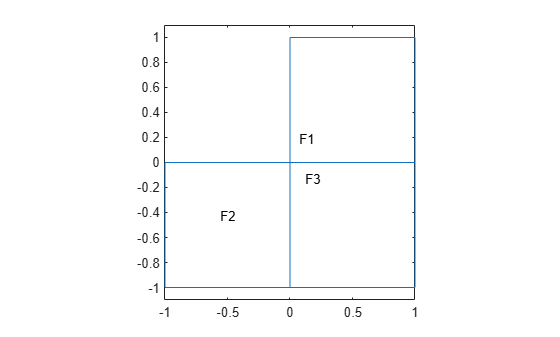findCoefficients

Locate active PDE coefficients

Description

example

CA = findCoefficients(coeffs,RegionType,RegionID) returns the active coefficient assignment CA for the coefficients in the specified region.

Examples

collapse all

Create a PDE model that has a few subdomains.

model = createpde();
geometryFromEdges(model,@lshapeg);
pdegplot(model,'FaceLabels','on')
ylim([-1.1,1.1])
axis equalSet coefficients on each pair of regions.

specifyCoefficients(model,'m',0,'d',0,'c',12,'a',0,'f',1,'Face',[1,2]);
specifyCoefficients(model,'m',0,'d',0,'c',13,'a',0,'f',2,'Face',[1,3]);
specifyCoefficients(model,'m',0,'d',0,'c',23,'a',0,'f',3,'Face',[2,3]);

Check the coefficient specification for region 1.

coeffs = model.EquationCoefficients;
ca = findCoefficients(coeffs,'Face',1)
ca =
CoefficientAssignment with properties:

RegionType: 'face'
RegionID: [1 3]
m: 0
d: 0
c: 13
a: 0
f: 2

Input Arguments

collapse all

Model coefficients, specified as the EquationCoefficients property of a PDE model. Coefficients can be complex numbers.

Example: model.EquationCoefficients

Geometric region type, specified as 'Face' for a 2-D model, or 'Cell' for a 3-D model.

Example: ca = findCoefficients(coeffs,'Face',[1,3])

Data Types: char | string

Region ID, specified as a vector of positive integers. View the subdomain labels for a 2-D model using pdegplot(model,'FaceLabels','on'). Currently, there are no subdomains for 3-D models, so the only acceptable value for a 3-D model is 1.

Example: ca = findCoefficients(coeffs,'Face',[1,3])

Data Types: double

Output Arguments

collapse all

Coefficient assignment, returned as a CoefficientAssignment Properties object.

Introduced in R2016a

Partial Differential Equation Toolbox DocumentationGet trial now